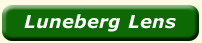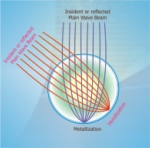General Description The Luneberg lens is a passive radar augmentation device used to increase the radar reflectivity of a target without the use of additional energy The lens reflector is a sphere in shape, usually composed of concentric dielectric shells. By the proper selection of dielectric constants for each shell, radar energy incident on one of the faces of the lens is focused at a point on the rear surface of the lens. The rear conductive surface reflects radar energy back to the source. Physical Characteristics: The physical Characteristics of the lens vary according to there application, as depicted above. The lens is available in several different diameters.The lens contains adapter brackets on the rear for mounting to any flat bulkhead. Basic Of the Luneberg Lens: The ideal Luneberg lens is sphere having an index of refraction varying from n =1 at the surface to n=2 at the center and is embedded in a medium whose index of refraction equals to that of the surface of the lens (e.g. n=1). The index varies from the center (r=0) to the surface(r=R) of the lens, where R is the radius of the lens, according to ε=2-(r/R)**2. The spherical shapes of the Luneberg lens in contrast to conventional lenses, has no intrinsic optical axis. For this reason, plane waves incident on the lens encounter an effective optical axis in the direction of the plan waves. This general condition yields a lens which operates similarly on plan waves incident from different directions. With the ideal index of the refraction above, the incident plane wave is focused on a point at the opposite surface of the lens.Principal of Operation: (1) Basically the Luneberg lens is made of concentric shell of polystyrene with varying reflective indices (dielectric constants) from 1 to 2, the highest refractive index being the innermost shell. (2) A ray passing through the various concentric shell will bend towards normal and then focus at a point. If a metal reflector is placed around the focal point, the ray gets reflected and retraces the same path. This is the principal of Luneberg Lenses (3) In order to have smooth bending of the ray theoretically, many concentric shells are required. In practice, a few concentric shell are used. (4)The radar cross section augmentation of the lens is given by the formula( 4∏3R4/4λ ) The RCS is inversely proportional to the squire of the wave length and directly proportional to the 4th power of the radius.